## Graph-theory

 Question 1

What is the maximum number of edges in an acyclic undirected graph with n vertices?

 A n - 1 B n C n + 1 D 2n - 1
Engineering-Mathematics       Graph-Theory       GATE 2004-IT
Question 1 Explanation:
Maximum number of edges in an acyclic undirected graph = No. of vertices - 1
= n - 1
 Question 2

What is the number of vertices in an undirected connected graph with 27 edges, 6 vertices of degree 2, 3 vertices of degree 4 and remaining of degree 3?

 A 10 B 11 C 18 D 19
Engineering-Mathematics       Graph-Theory       GATE 2004-IT
Question 2 Explanation:
Let x = Total no. of vertices
By Handshaking Lemma,
6 * 2 + 3 * 4 + (x - 9) * 3 = 27 * 2
24 + (x - 9) * 3 = 54
x = 19
 Question 3

Graph G is obtained by adding vertex s to K3,4 and making s adjacent to every vertex of K3,4. The minimum number of colours required to edge-colour G is _____.

 A 7
Engineering-Mathematics       Graph-Theory       GATE 2020       Video-Explanation
Question 3 Explanation:
In k3x4 there are two sets with sizes 3,4. (it is a complete bipartite graph).
The vertex in the set of size 3 has 4 edges connected to 4 vertices on other set. So, edge color of G is max(3,4) i.e. 4.
When a vertex is added to the graph with 7 vertices ( K3x4 has 7 vertices), there would be 7 edges associated to that new vertex. As per the edge coloring “no two adjacent edges have same color).
As the new vertex with 7 edges need to be colored with 7 colors, the edge color of graph G is 7.
 Question 4
Consider a simple undirected graph of 10 vertices. If the graph is disconnected, then the maximum number of edges it can have is ____________.
 A 36
Engineering-Mathematics       Graph-Theory       GATE 2022       Video-Explanation
Question 4 Explanation:
We obtain maximum number of edges in a disconnected graph, with one isolated vertex and remaining completely connected.
Given 10 vertices,
Then we can have a complete graph with 9 vertices and one isolated verted.
Number of edges in complete graph with 9 edges is n(n-1)/2 = 9*8/2 = 36
 Question 5
Consider a simple undirected unweighted graph with at least three vertices. If A is the adjacency matrix of the graph, then the number of 3-cycles in the graph is given by the trace of
 A A ^3 B A^ 3 divided by 2 C A ^3 divided by 3 D A ^3 divided by 6
Engineering-Mathematics       Graph-Theory       GATE 2022       Video-Explanation
 Question 6
The following simple undirected graph is referred to as the Peterson graph.Which of the following statements is/are TRUE?
 A The chromatic number of the graph is 3. B The graph has a Hamiltonian path C The following graph is isomorphic to the Peterson graph.D The size of the largest independent set of the given graph is 3. (A subset of vertices of a graph form an independent set if no two vertices of the subset are adjacent.)
Engineering-Mathematics       Graph-Theory       GATE 2022       Video-Explanation
Question 6 Explanation:
Chromatic number is 3.Peterson graph ihas hamiltonian path but not hamiltonian cycle
Given graph in option C is isomorphic as the given has same number of vertice, edges, degree sequence and cycles.
LArgest independent set can be more than 3Question 7
Which of the properties hold for the adjacency matrix A of a simple undirected unweighted graph having n vertices?
 A The diagonal entries of A 2 are the degrees of the vertices of the graph. B If the graph is connected, then none of the entries of A^ n + 1 + I n can be zero. C If the sum of all the elements of A is at most 2( n- 1), then the graph must be acyclic. D If there is at least a 1 in each of A ’s rows and columns, then the graph must be Connected.
Engineering-Mathematics       Graph-Theory       GATE 2022       Video-Explanation
Question 7 Explanation:
IF A is adjacency matrix, then in the matrix A*A = A^2 we have
The entries aii show the number of 2-length paths between the nodes i and j. Why this happens is easy to see: if there is an edge ij and an edge jk, then there will be a path ik through j. The entries ii are the degrees of the nodes i.
Similarly in A^3 we have the entries aii that show the number of 3-length paths between the nodes i and j.
In A^n-1 + I n, we will have at least n-1 length paths, so there is no possibility of zero entires
 Question 8

Let G be a graph with 100 vertices numbered 1 to 100. Two vertices i and j are adjacent if |i - j| = 8 or |i - j| = 12. The number of connected components in G is

 A 8 B 4 C 12 D 25
Engineering-Mathematics       Graph-Theory       GATE 1997
Question 8 Explanation:
From the description, it is clear that vertices are connected as follows:
1 — 9 — 17 — ......... — 97
2 — 10 — 18 — ......... — 98
3 — 11 — 19 — ......... — 99
4 — 12 — 20 — ......... — 100
5 — 13 — 21 — ......... — 93
6 — 14 — 22 — ......... — 94
7 — 15 — 23 — ......... — 95
8 — 16 — 24 — ......... — 96
We have covered all vertices using 8 vertex sets considering only |i - j| = 8. Using |i - j| = 12 we can see the vertex 1 is connected to 13, 2 to 14, 3 to 15 and 4 to 16. So the top 4 vertex sets are infact connected to the bottom 4 sets, thus reducing the connected components to 4.
 Question 9

(a) Prove by induction that the expression for the number of diagonals in a polygon of n sides is n(n-3)/2.

(b) Let R be a binary relation on A = {a, b, c, d, e, f, g, h} represented by following two component digraph. Find the smallest integers m and n such that mm = Rn.A Theory Explanation.
Engineering-Mathematics       Graph-Theory       GATE 1998
 Question 10

Let (A, *) be a semi group. Furthermore, for every a and b in A, if a ≠ b, then a*b ≠ b*a.

(a) Show that for every a in A

` a*a = a `

(b) Show that for every a, b in A

` a*b*a = a  `

(c) Show that for every a, b, c in A

` a*b*c = a*c `
 A Theory Explanation.
Engineering-Mathematics       Graph-Theory       GATE 1998
 Question 11

Let G be a connected, undirected graph. A cut in G is a set of edges whose removal results in 0 being broken into two or more components which are not connected with each other. The size of a cut is called its cardinality. A men-cut of G is a cut in G of minimum cardinality. Consider the following graph.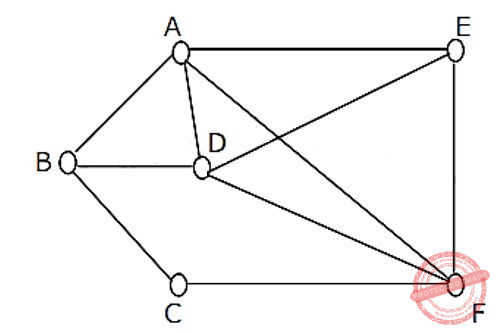(a) Which of the following sets of edges is a cut?
(i) {(A,B), (E,F), (B,D), (A,E), (A,D)}
(ii) {(B,D), (C,F), (A,B)}

(b) What is the cardinality of a min-cut in the graph?

(c) Prove that if a connected undirected graph G with n vertices has a min-cut of cardinality K, then G has atleast (nk/2) edges.

 A Theory Explanation.
Engineering-Mathematics       Graph-Theory       GATE 1999
 Question 12

Let S be a set of n elements {1, 2, …., n} and G a graph with 2n vertices, each vertex corresponding to a distinct subset of S. Two vertices are adjacent iff the symmetric difference of the corresponding sets has exactly 2 elements. Note: The symmetric difference of two sets R1 and R2 is defined as (R1/R2)∪(R2/R1)
(a) Every vertex in G has the same degree. What is the degree of a vertex in G?
(b) How many connected components does G have?

 A Theory Explanation is given below.
Engineering-Mathematics       Graph-Theory       GATE 2000
 Question 13

The minimum number of colours required to colour the vertices of a cycle with η nodes in such a way that no two adjacent nodes have the same colour is

 A 2 B 3 C 4 D n - 2[n/2] + 2
Engineering-Mathematics       Graph-Theory       GATE 2002
Question 13 Explanation:
We need 2 colours to colour even cycle and 3 colours to colour odd cycle.
 Question 14

Maximum number of edges in a n-node undirected graph without self loops is

 A n2 B n(n-1)/2 C n-1 D (n+1)(n)/2
Engineering-Mathematics       Graph-Theory       GATE 2002
Question 14 Explanation:
The set of vertices has size n, the number of such subsets is given by the binomial coefficient C(n, 2)⋅ C(n, 2) = n(n-1)/2.
 Question 15

Let G be an arbitrary graph with n nodes and k components. If a vertex is removed from G, the number of components in the resultant graph must necessarily lie between

 A k and n B k – 1 and k + 1 C k – 1 and n – 1 D k + 1 and n – k
Engineering-Mathematics       Graph-Theory       GATE 2003
Question 15 Explanation:
While a vertex is removed from a graph then that can be itself be forms a new component. The minimum number of components is k-1.
If a vertex is removed then it results that all the components are also be disconnected. So removal can create (n-1) components.
 Question 16

How many perfect matching are there in a complete graph of 6 vertices ?

 A 15 B 24 C 30 D 60
Engineering-Mathematics       Graph-Theory       GATE 2003
Question 16 Explanation:
We have formula to find no. of perfect matching in complete graphs of 2n vertices,
(2n)!/n!×2n
Given, 2n = 6 ⇒ n = 3
So, finally, 6!/3!×23 = 15
 Question 17

A graph G = (V,E) satisfies |E|≤ 3|V|-6. The min-degree of G is defined as. Therefore, min-degree of G cannot be

 A 3 B 4 C 5 D 6
Engineering-Mathematics       Graph-Theory       GATE 2003
Question 17 Explanation:
The minimum degree of G = minv∈V {degree(v)}
|E| ≤ 3|v| - 6
Based on handshaking lemma, the minimum degree is (min×|v|)/2
⇒ (min×|v|)/2 ≤ 3|v| - 6
Checking the options lets take min=6
(6×|v|)/2 ≤ 3|v| - 6
0 ≤ -6 (Not satisfied)
And which is inconsistent.
 Question 18

The minimum number of colours required to colour the following graph, such that no two adjacent vertices are assigned the same colour, is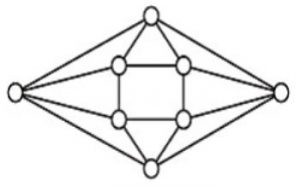A 2 B 3 C 4 D 5
Engineering-Mathematics       Graph-Theory       GATE 2004
Question 18 Explanation:→ a, b, c, d = 4
→ The minimum no. of colours required to colour a graph = 4 (no two adjacent vertices have same colours)
 Question 19

How many graphs on n labeled vertices exist which have at least (n2 - 3n)/2 edges?

 AB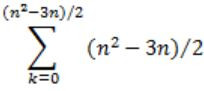C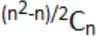D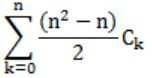Engineering-Mathematics       Graph-Theory       GATE 2004
Question 19 Explanation:
No. of atleast edges = (n2-3n)/2 = e
Maximum no. of vertices = n(n-1)/2 = v
No. of graphs with minimum b edges is
= C(v,e) + C(v,e+1) + C(v,e+2) + ... + C(v,v)
= C((v,v-e) + C(v,v-(e+1)) + C(v,v-(e+2)) + ... + C(v,0)
= C(a,n) + C(a,n-1) + C(a,n-2) + ... + C(a,0) (since a-b=n)
= C(n(n-1)/2,n) + C(n(n-1)/2,n-1) + ... + C(n(n-1)/2,0)Question 20

Let G1 = (V,E1) and G2 = (V,E2) be connected graphs on the same vertex set V with more than two vertices. If G1 ∩ G2 = (V, E1 ∩ E2) is not a connected graph, then the graph G1 U G2 = (V, E1 U E2)

 A cannot have a cut vertex B must have a cycle C must have a cut-edge (bridge) D has chromatic number strictly greater than those of G1 and G2
Engineering-Mathematics       Graph-Theory       GATE 2004
Question 20 Explanation:
Lets try to take counter example for each of them,
(A)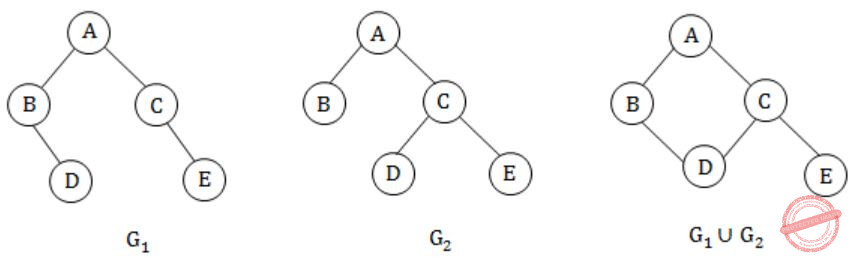False, since in G1∪G2 'C' is a cut vertex.
(B) True, for all conditions.
(C)False. G1∪G2 has no bridge.
D)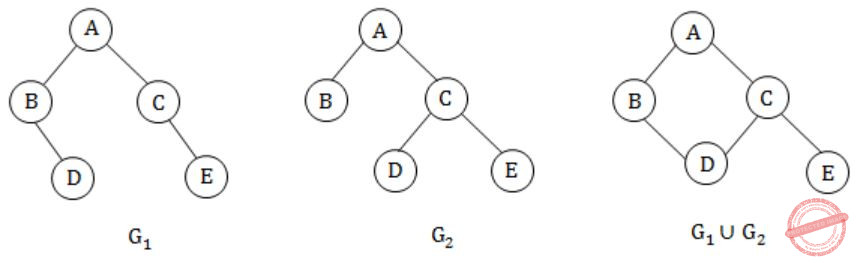False. G1∪G2, G1, G2 all the three graphs have chromatic number of 2.
 Question 21

Let G be a simple connected planar graph with 13 vertices and 19 edges. Then, the number of faces in the planar embedding of the graph is:

 A 6 B 8 C 9 D 13
Engineering-Mathematics       Graph-Theory       GATE 2005
Question 21 Explanation:
No. of faces in a planar embedding of a graph is
F = E - V + 2 [From Euler's formula i.e., F + V - E = 2]
F = 19 - 13 +2
F = 8
 Question 22

Let G be a simple graph with 20 vertices and 100 edges. The size of the minimum vertex cover of G is 8. Then, the size of the maximum independent set of G is

 A 12 B 8 C Less than 8 D More than 12
Engineering-Mathematics       Graph-Theory       GATE 2005
Question 22 Explanation:
No. of vertices = 20
Edges = 100
Minimum cover of vertex G is = 8
Maximum Independent set of G = No. of vertices - Minimum cover of vertex G
= 20 - 8
= 12
 Question 23

Which one of the following graphs is NOT planar?A G1 B G2 C G3 D G4
Engineering-Mathematics       Graph-Theory       GATE 2005
Question 23 Explanation:
G2 can also be drawn aswhich is planar
G3 can also be drawn aswhich is planar
G4 can also be drawn as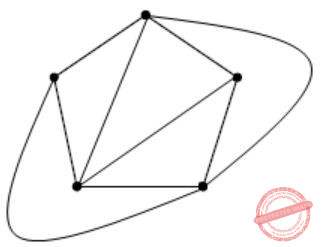which is planar
But G1 cannot be drawn as planar graph.
Hence, option (A) is the answer.
 Question 24

The 2n vertices of a graph G corresponds to all subsets of a set of size n, for n ≥ 6. Two vertices of G are adjacent if and only if the corresponding sets intersect in exactly two elements.

The number of vertices of degree zero in G is:

 A 1 B n C n+1 D 2n
Engineering-Mathematics       Graph-Theory       GATE 2006
Question 24 Explanation:
No. of vertices with degree zero is
= no. of subsets with size less than or equal to 1
= n+1, because in question it is given that the two vertices are connected if and only if the corresponding sets intersect in exactly two elements.
 Question 25

The 2n vertices of a graph G corresponds to all subsets of a set of size n, for n ≥ 6. Two vertices of G are adjacent if and only if the corresponding sets intersect in exactly two elements.

The maximum degree of a vertex in G is:

 AB 2n-2 C 2n-3 × 3 D 2n-1
Engineering-Mathematics       Graph-Theory       GATE 2006
Question 25 Explanation:
The degree of each subset with k elements will be
(k(c))2 2n-k
∴ We need to find 'k' value such that, the value will be maximum.[k should be an integer].
If you differentiate (k(c))2 2n-k w.r.t. k and equal to 0.
You will get k = 2/(loge)2 which is not an integer.
So you can see it like∴ The maximum degree 3⋅2n-3 at k=3 or k=4.
 Question 26

The 2n vertices of a graph G corresponds to all subsets of a set of size n, for n ≥ 6. Two vertices of G are adjacent if and only if the corresponding sets intersect in exactly two elements.

The number of connected components in G is:

 A n B n+2 C 2n/2 D 2n / n
Engineering-Mathematics       Graph-Theory       GATE 2006
Question 26 Explanation:
Not connected nodes is n+1.
While other nodes are connected so that total number of connected components is (n+1)+1
(here we are adding 1 because it is connected corresponding remaining vertices)
= n+2
 Question 27

The minimum number of edges in a connected cyclic graph on n vertices is:

 A n - 1 B n C n + 1 D None of the above
Engineering-Mathematics       Graph-Theory       GATE 1995
Question 27 Explanation:
In a normal graph number of edges required for n vertices is n-1, and in cyclic graph it is n.
In cyclic graph:
No. of edges = No. of vertices
⇒ n = n
 Question 28

Prove that in finite graph, the number of vertices of odd degree is always even.

 A Theory Explanation.
Engineering-Mathematics       Graph-Theory       GATE 1995
 Question 29

The number of distinct simple graphs with upto three nodes is

 A 15 B 10 C 7 D 9
Engineering-Mathematics       Graph-Theory       GATE 1994
Question 29 Explanation:Question 30

The number of edges in a regular graph of degree d and n vertices is _________.

 A d*n/2
Engineering-Mathematics       Graph-Theory       GATE 1994
Question 30 Explanation:
Sum of degree of vertices = 2 × no. of edges
d * n = 2 * |E|
∴ |E| = d*n/2
 Question 31

Let G be a weighted undirected graph and e be an edge with maximum weight in G. Suppose there is a minimum weight spanning tree in G containing the edge e. Which of the following statements is always TRUE?

 A There exists a cutset in G having all edges of maximum weight B There exists a cycle in G having all edges of maximum weight C Edge e cannot be contained in a cycle D All edges in G have the same weight
Algorithms       Graph-Theory       GATE 2005-IT
Question 31 Explanation:
(A) True, because if there is heaviest edge in MST, then there exist a cut with all edges with weight equal to heaviest edge.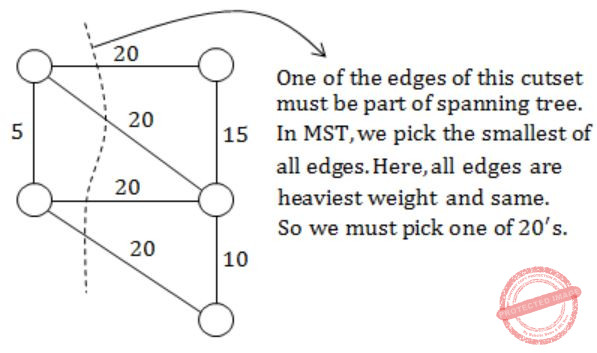(B) False, because the cutset of heaviest edge may contain only one edge.
(C) False. The cutset may form cycle with other edge.
(D) False. Not always true.
 Question 32

Let G be a directed graph whose vertex set is the set of numbers from 1 to 100. There is an edge from a vertex i to a vertex j iff either j = i + 1 or j = 3i. The minimum number of edges in a path in G from vertex 1 to vertex 100 is

 A 4 B 7 C 23 D 99
Algorithms       Graph-Theory       GATE 2005-IT
Question 32 Explanation:
Edge set consists of edges from i to j, using either
j = i +1
(or)
j = 3i
Second option will help us reach from 1 to 100 rapidly. The trick to solve this question is to think in reverse way. Instead of finding a path from 1 to 100, try to find a path from 100 to 1.
So, the edge sequence with minimum number of edges is
1 → 3 → 9 → 10 → 11 → 33 → 99 → 100
which consists of 7 edges.
 Question 33
In an undirected connected planar graph G, there are eight vertices and five faces. The number of edges in G is ______
 A 11
Engineering-Mathematics       Graph-Theory       GATE 2021 CS-Set-1       Video-Explanation
Question 33 Explanation:

v - e + f = 2

v is number of vertices

e is number of edges

f is number of faces including bounded and unbounded

8-e+5=2

=> 13-2 =e

The number of edges  are =11

 Question 34
Let G = (V, E) be an undirected unweighted connected graph. The diameter of G is defined as:Let M be the adjacency matrix of G.
Define graph G2on the same set of vertices with adjacency matrix N, whereWhich one of the following statements is true?
 ABCDEngineering-Mathematics       Graph-Theory       GATE 2021 CS-Set-1       Video-Explanation
Question 34 Explanation:Question 35

What is the chromatic number of the following graph?A 2 B 3 C 4 D 5
Engineering-Mathematics       Graph-Theory       GATE 2008-IT
Question 35 Explanation:
Chromatic number = 3
→ Chromatic number of a graph is the smallest number of colours needed to colour the vertices so that no two adjacent vertices share the same colour.Question 36

What is the size of the smallest MIS(Maximal Independent Set) of a chain of nine nodes?

 A 5 B 4 C 3 D 2
Engineering-Mathematics       Graph-Theory       GATE 2008-IT
Question 36 Explanation:
1 - 2 - 3 - 4 - 5 - 6 - 7 - 8 - 9
(2, 5, 8) is the maximal independent set for a chain of 9 nodes. If we add any start node to the set then it will not be MIS.
Independent set:
A set of vertices is called independent set such that no two vertices in the set are adjacent.
 Question 37

G is a simple undirected graph. Some vertices of G are of odd degree. Add a node v to G and make it adjacent to each odd degree vertex of G. The resultant graph is sure to be

 A Regular B Complete C Hamiltonian D Euler
Engineering-Mathematics       Graph-Theory       GATE 2008-IT
Question 37 Explanation:
Euler graph theory is a trail in a finite graph which visits every edge exactly once and which is a undirected graph.
→ In Euler graph all degrees must be even for all nodes. And number of odd degree vertices should be even.
→ So, degree of this new node will be even and as a new edge is formed between this new node and all other nodes of odd degree hence here is not a single node exists with degree odd so this is Euler graph.
 Question 38

Consider the undirected graph G defined as follows. The vertices of G are bit strings of length n. We have an edge between vertex u and vertex v if and only if u and v differ in exactly one bit position (in other words, v can be obtained from u by flipping a single bit). The ratio of the chromatic number of G to the diameter of G is

 A 1/(2n-1) B 1/n C 2/n D 3/n
Engineering-Mathematics       Graph-Theory       GATE 2006-IT
Question 38 Explanation:
For the given condition we can simply design a K-map and mark an edge between every two adjacent cells in K-map.
That will give us a bipartite graph, with chromatic number = 2
Also from the same we can conclude that we need for a 'n' bit string, to traverse no more than (n-1) edges or 'n' vertices to get a path between two arbitrary points. So the ratio is (2/n).
 Question 39

Which of the following graphs is/are planner?A Theory Explanation is given below.
Engineering-Mathematics       Graph-Theory       GATE 1989       Video-Explanation
Question 39 Explanation:
(i) G1 is K33 which is planar graph with the minimum number of edges.
→ Let us assume K33is a planar graph. Then it satisfy the useful corollary. As there is no triangle in K33.
Let G be a connected planar graph with n vertices and m edges, and no triangles. Then m≤2n-4.
Where m=9, n=6
⇒ 9 ≤ 12 - 4
⇒ 9 ≤ 8, which is to be false, then K33 is non-planar graph.
(ii) G2 is a planar graph. Because it can be redrawn like as below.(iii) Let us assume G3 be a planar graph then it also be satisfy useful corollary.
Where m=9, n=6
then 9 ≤ 12-4
9 ≤ 8 is False
So, G3 is non-planar graph.
Answer: Only G2 is planar graph.
 Question 40

Choose the correct alternatives (More than one may be correct).

A graph is planar if and only if,
 A It does not contain subgraphs homeomorphic to k5 and k3,3. B It does not contain subgraphs isomorphic to k5 or k3,3. C It does not contain a subgraph isomorphic to k5 or k3,3. D It does not contain a subgraph homeomorphic to k5 or k3,3.
Engineering-Mathematics       Graph-Theory       GATE 1990       Video-Explanation
Question 40 Explanation:
A graph is non-planar if and only if it contains a subgraph which is homomorphic to k5or k3,3. This is kuratowshi theorem.
 Question 41

The maximum number of possible edges in an undirected graph with a vertices and k components is ________.

 A (n-k)(n-k+1)/2
Engineering-Mathematics       Graph-Theory       GATE 1991       Video-Explanation
Question 41 Explanation:
N vertices and K components.
To get maximum, take one vertex each for each component, except last component.
Now, k-1 components have 1 vertex each and so on edges.
The last component has (n-(k-1)) vertices. So make the last component complete, i.e., it has n-(n-1)C2
= (n-k)(n-k+1)/2
= maximum no. of edges
 Question 42

Maximum number of edges in a planar graph with n vertices is ________

 A 3n - 6
Engineering-Mathematics       Graph-Theory       GATE 1992       Video-Explanation
Question 42 Explanation:
The maximum is 3(n - 8) for every n>2.
⇒ (3n - 2) = 3n - 6
 Question 43

A non-planar graph with minimum number of vertices has

 A 9 edges, 6 vertices B 6 edges, 4 vertices C 10 edges, 5 vertices D 9 edges, 5 vertices
Engineering-Mathematics       Graph-Theory       GATE 1992       Video-Explanation
Question 43 Explanation:The above graph with 5 vertices and 10 edges is non-planar.
 Question 44

Let T be a Depth First Tree of a undirected graph G. An array P indexed by vertices of G is given. P[V] is the parent of vertex V, in T. Parent of the root is the root itself.

Give a method for finding and printing the cycle formed if the edge (u,v) of G not in T (i.e., e ∈ G − T) is now added to T.

Time taken by your method must be proportional to the length of the cycle.

Describe the algorithm in a PASCAL – like language. Assume that the variables have been suitably declared.

 A Theory Explanation.
Engineering-Mathematics       Graph-Theory       GATE 1992       Video-Explanation
 Question 45

(a) If G is a group of even order, then show that there exists an element a ≠ e, the identity in g, such that a2 = e

(b) Consider the set of integers {1,2,3, 4,6,8,12,24} together with the two binary operations LCM (lowest common multiple) and GCD (greatest common divisor). Which of the following algebraic structures does this represent?

``` (i) group            (ii) ring
(iii) field          (iv) lattice  ```
 A Theory Explanation.
Engineering-Mathematics       Graph-Theory       GATE 1992       Video-Explanation
 Question 46

Let G be the non-planar graph with the minimum possible number of edges. Then G has

 A 9 edges and 5 vertices B 9 edges and 6 vertices C 10 edges and 5 vertices D 10 edges and 6 vertices
Engineering-Mathematics       Graph-Theory       GATE 2007
Question 46 Explanation:
Using Euler’s formula we know that,
if n ≥ 3 then e ≤ 3n-6 (for planarity)
where n = no. of vertices
e = no. of edges
Now lets check the options.
A) e=9, n=5
9 ≤ 3(5) - 6
9 ≤ 15 - 6
9 ≤ 9
Yes, it is planar.
B) e=9, n=6
9 ≤ 3(6) - 6
9 ≤ 18 - 6 9 ≤ 12 Yes, it is planar.
iii) e=10, n=5
10 ≤ 3(5) - 6
10 ≤ 15 - 6
10 ≤ 9 No, it is not planar.
So, option C is non-planar graph.
iv) e=10, n=6
10 ≤ 3(6) - 6
10 ≤ 18 - 6
10 ≤ 12
Yes, it is planar.
 Question 47

Which of the following graphs has an Eulerian circuit?

 A Any k-regular graph where k is an even number. B A complete graph on 90 vertices. C The complement of a cycle on 25 vertices. D None of the above.
Engineering-Mathematics       Graph-Theory       GATE 2007
Question 47 Explanation:
Two necessary condition for the existence of Eulerian circuits is
→ all vertices in the graph have an "even degree".
→ And the graph must be corrected.
Now in option (C) it is saying that the complement of a cycle on 25 vertices without complement the degree of each vertex is 2.
Now since there are 25 vertices, so maximum degree of each vertex will be 24 and so in complement of cycle each vertex degree will be 24 - 2 = 22.
There is a theorem which says "G be a graph with n vertices and if every vertex has a degree of atleast n-1/2 then G is connected."
So we can say that complement of cycle with 25 vertices fulfills both the conditions, and hence is Eulerian circuit.
 Question 48

Which of the following statements is true for every planar graph on n vertices?

 A The graph is connected B The graph is Eulerian C The graph has a vertex-cover of size at most 3n/4 D The graph has an independent set of size at least n/3
Engineering-Mathematics       Graph-Theory       GATE 2008
Question 48 Explanation:
Lets do with elimination method,
(A) Consider the following disconnected graph which is planar.So false.
(B) A graph is Eulerian if all vertices have even degree but a planar graph can have vertices with odd degree.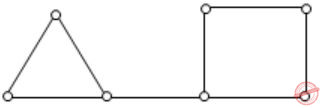So false.
(D) Consider K4 graph. It has independent set size 1 which is less than 4/3.So false.
Hence, option (C) is correct.
 Question 49

In a connected graph, a bridge is an edge whose removal disconnects a graph. Which one of the following statements is true?

 A A tree has no bridges B A bridge cannot be part of a simple cycle C Every edge of a clique with size 3 is a bridge (A clique is any complete sub graph of a graph) D A graph with bridges cannot have a cycle
Engineering-Mathematics       Graph-Theory       GATE 2015 [Set-2]       Video-Explanation
Question 49 Explanation:
Since, every edge in a tree is bridge
∴ (A) is false
Since, every edge in a complete graph kn(n≥3) is not a bridge ⇒
(C) is false
Let us consider the following graph G:This graph has a bridge i.e., edge ‘e’ and a cycle of length ‘3’
∴ (D) is false
Since, in a cycle every edge is not a bridge
∴ (B) is true
 Question 50

A graph is self-complementary if it is isomorphic to its complement for all self-complementary graphs on n vertices, n is

 A A multiple of 4 B Even C Odd D Congruent to 0 mod 4, or, 1 mod 4
Engineering-Mathematics       Graph-Theory       GATE 2015 [Set-2]       Video-Explanation
Question 50 Explanation:
An n vertex self-complementary graph has exactly half number of edges of the complete graph i.e., n(n-1)/4 edges. Since n(n – 1) must be divisible by 4, n must be congruent to 0 or 1 module 4.
 Question 51

The minimum number of colours that is sufﬁcient to vertex-colour any planar graph is ________.

 A 4 B 5 C 6 D 7
Engineering-Mathematics       Graph-Theory       GATE 2016 [Set-2]       Video-Explanation
Question 51 Explanation:
The 4-colour theorem of the planar graph describes that any planar can atmost be colored with 4 colors.
Here it is asked about the sufficient number of colors, so with the worst case of 4 colors we can color any planar graph.
 Question 52

G is an undirected graph with n vertices and 25 edges such that each vertex of G has degree at least 3. Then the maximum possible value of n is ___________.

 A 16 B 17 C 18 D 19
Engineering-Mathematics       Graph-Theory       GATE 2017 [Set-2]       Video-Explanation
Question 52 Explanation:
An undirected graph ‘G’ has ‘n’ vertices & 25 edges.
Degree of each vertex ≥ 3|v| = 2|E|
The relation between max and min degree of graph are
m ≤ 2|E| / |v| ≤ M
Given minimum degree = 3
So, 3 ≤2 |E| / |v|
3|v| ≤ 2|E|
3(n) ≤ 2(25)
n ≤ 50/3
n ≤ 16.6
(n = 16)
 Question 53

How many undirected graphs (not necessarily connected) can be constructed out of a given set V = {v1, v2, ...,vn} of n vertices?

 A n(n-1)/2 B 2n C n! D 2n(n-1)/2
Engineering-Mathematics       Graph-Theory       GATE 2001
Question 53 Explanation:
With n vertices no. of possible edges = n C 2
Each subset of these edges will be form a graph.
No. of possible undirected graphs is 2(n C 2)
⇒ 2(n(n-1)/2)
 Question 54

Let G be an undirected complete graph on n vertices, where n > 2. Then, the number of different Hamiltonian cycles in G is equal to

 A n! B 1 C (n-1)! D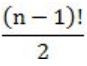Engineering-Mathematics       Graph-Theory       GATE 2019       Video-Explanation
Question 54 Explanation:
A Hamiltonian cycle is a closed loop on a graph where every node (vertex) is visited exactly once.
The total number of hamiltonian cycles in a complete graph are
(n-1)!/2, where n is number of vertices.
 Question 55

Let G be a connected planar graph with 10 vertices. If the number of edges on each face is three, then the number of edges in G is _______________.

 A 24 B 25 C 26 D 27
Engineering-Mathematics       Graph-Theory       GATE 2015 [Set-1]       Video-Explanation
Question 55 Explanation:
By Euler’s formula,
|V| + |R| = |E| + 2 ------(1) where |V|, |E|, |R| are respectively number of vertices, edges and faces (regions)
Given |V| = 10 ------(2) and number of edges on each face is three
∴3|R| = 2|E| ⇒ |R| = 2/3|E| ------(3)
Substituting (2), (3) in (1), we get
10 + 2/3|E| = |E| + 2 ⇒ |E|/3 = 8 ⇒ |E| = 24
 Question 56
Let G be a simple, finite, undirected graph with vertex set {v1,...,vn}. Let Δ(G) denote the maximum degree of G and let N = {1, 2,...} denote the set of all possible colors. Color the vertices of G using the following greedy strategy: for i = 1,...,n
color(vi) ← min{j ε N : no neighbour of vi is colored j}
Which of the following statements is/are TRUE?
 A This procedure results in a proper vertex coloring of G. B The number of colors used is at most Δ(G) + 1. C The number of colors used is at most Δ(G). D The number of colors used is equal to the chromatic number of G.
Engineering-Mathematics       Graph-Theory       GATE 2023       Video-Explanation
 Question 57

Let G = (V,E) be a directed graph where V is the set of vertices and E the set of edges. Then which one of the following graphs has the same strongly connected components as G?

 A G1=(V,E1) where E1={(u,v)|(u,v)∉E} B G2=(V,E2 )where E2={(u,v)│(u,v)∈E} C G3=(V,E3) where E3={(u,v)|there is a path of length≤2 from u to v in E} D G4=(V4,E) where V4 is the set of vertices in G which are not isolated
Engineering-Mathematics       Graph-Theory       GATE 2014 [Set-1]       Video-Explanation
Question 57 Explanation:
G(V, E) is a directed graph.
→ It strongly connected.
(A) G1=(V,E1) where E1={(u,v)|(u,v)∉E}
If (u, v) does not belong to the edge set ‘E’, then it indicates there are no edges. So, it is not connected.
(B) G2=(V,E2 )where E2={(u,v)│(u,v)∈E}
Given that ‘G’ is directed graph, i.e., it has path from each vertex to every other vertex.
Though direction is changed from (u, v) to (v, u), it is still connected component same as ‘G’.
(C) G3=(V,E3) where E3={(u,v)|there is a path of length≤2 from u to v in E}
This can also be true.
eg:Both from each vertex to other vertex is also exists. So it is also strongly connected graph.
(D) G4=(V4,E) where V4 is the set of vertices in G which are not isolated.
If ‘G’ has same ‘x’ no. of isolated vertices, one strongly connected component
then no. of SCC = x + 1
G4 contain only ‘1’ component, which is not same as G.
 Question 58

Consider an undirected graph where self-loops are not allowed. The vertex set of G is {(i,j): 1 ≤ i ≤ 12, 1 ≤ j ≤ 12}. There is an edge between (a,b) and (c,d) if |a - c| ≤ 1 and |b - d| ≤ 1. The number of edges in this graph is __________.

 A 506 B 507 C 508 D 509
Engineering-Mathematics       Graph-Theory       GATE 2014 [Set-1]       Video-Explanation
Question 58 Explanation:
The total number of vertices in the graph is 12*12 = 144. The vertices are allowed to connect in both horizontal and vertical directions which are separated by at most 1 distance.
If we observe the graph, it looks like a 12 by 12 grid. Each corner vertex has a degree of 3 and we have 4 corner vertices. 40 external vertices of degree 5 and remaining 100 vertices of degree 8.
From Handshaking theorem, sum of the degrees of the vertices is equal to the 2*number of edges in the graph.
⇒ (4*3) + (40*5) + (100*8) = 2*E
⇒ 1012 = 2*E
⇒ E = 506
 Question 59

An ordered -tuple (d1, d2, ..., dn) with d1 ≥ d2 ≥ ... dn is called graphic if there exists a simple undirected graph with n vertices having degrees d1, d2, ..., dn respectively. Which of the following 6-tuples is NOT graphic?

 A (1, 1, 1, 1, 1, 1) B (2, 2, 2, 2, 2, 2) C (3, 3, 3, 1, 0, 0) D (3, 2, 1, 1, 1, 0)
Engineering-Mathematics       Graph-Theory       GATE 2014 [Set-1]       Video-Explanation
Question 59 Explanation:
This can be checked by Havel-hakimi theorem:
A) (1, 1, 1, 1, 1, 1)Yes, it is a graph.
We will see that option (C) is not graphic.
 Question 60

The chromatic number of the following graph is _______.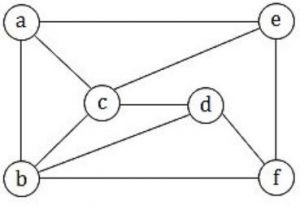A 1 B 2 C 3 D 4
Engineering-Mathematics       Graph-Theory       GATE 2018       Video-Explanation
Question 60 Explanation:
Chromatic number of the following graph is “3”There are 60 questions to complete.

Register Now